# How to Calculate Number of Tiles for different Spaces

If you are planning the type, cost and number of tiles calculation for your new home, there are simple techniques to do so. You will have to do some elementary study about your needs and availability to arrive at the right choice that fits into your budget.

## Type of Project

The steps to calculate the number of tiles remain the same irrespective of whether you are calculating for a floor or a wall. At a very elementary level, you just need a measuring tape, a notepad, a pen and a calculator (unless you can quickly compute numbers in your head!).

A few calculations and then you will be done with estimating the number and cost of the tiles that you select for your house.

## Factors to Be Considered

Wall tiles are in vogue right now and are being used in stylish and innovative ways all around us. Tiles are the foremost choice in interior decoration because of their versatility, longevity and affordability.

The tile industry continues to evolve and when you calculate the number of tiles for different spaces, there are multiple options available at your disposal. You must consider several factors if you want to choose the tile best suited for your needs:

### Location

Location is key in the selection process. You may like to install larger tiles in bigger spaces and areas that should have a majestic look. So, the total number of tiles will be proportionately calculated.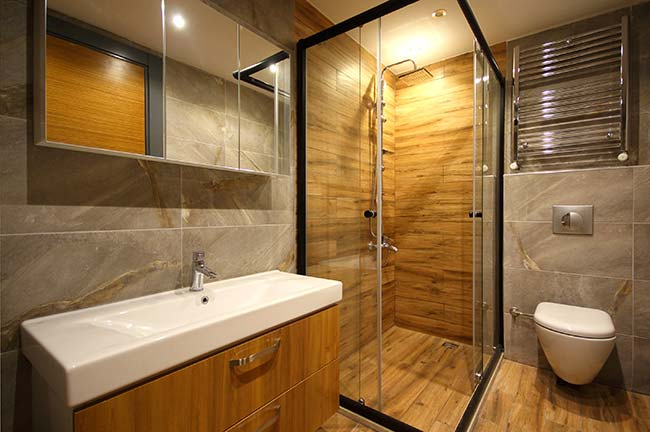### Design & Patterns

The decision has multiple implications. Some designs may be available only for large or small tiles or for tiles of differing quality depending on the application. This can impact the designs and patterns available to choose from.

If you choose high gloss tiles   for your bedroom, you can explore more combinations of patterns and colours. But high gloss tiles may not be the right fit for the portico where you would want something more resistant to slipping and sturdy.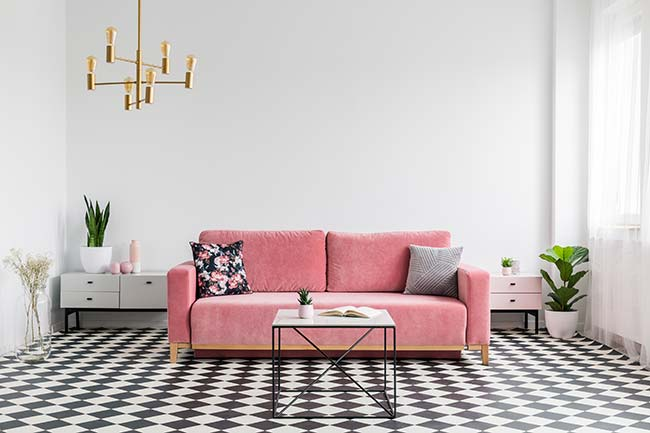### Interior Theme

theme is based on the optimum usage of the space and light in your residence or commercial place. Your interior theme will decide the design, pattern, finish and quantity of tiles you will use. Also, keep a surplus of 5-10% after your estimates as spare for future use.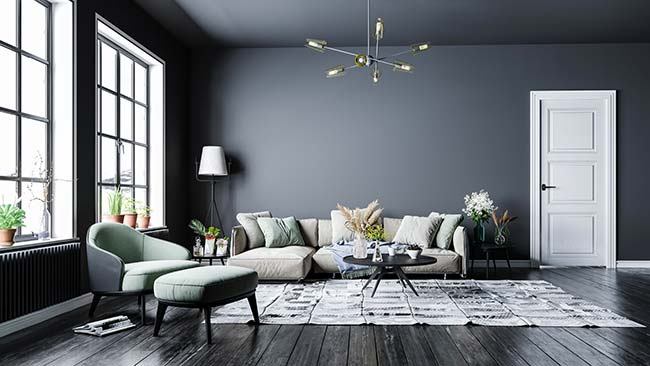### Size

This is, of course, the most critical direct factor having a bearing on the number of tiles needed. Once you have selected the tile and have the room dimensions handy, the number of tiles is just simple maths.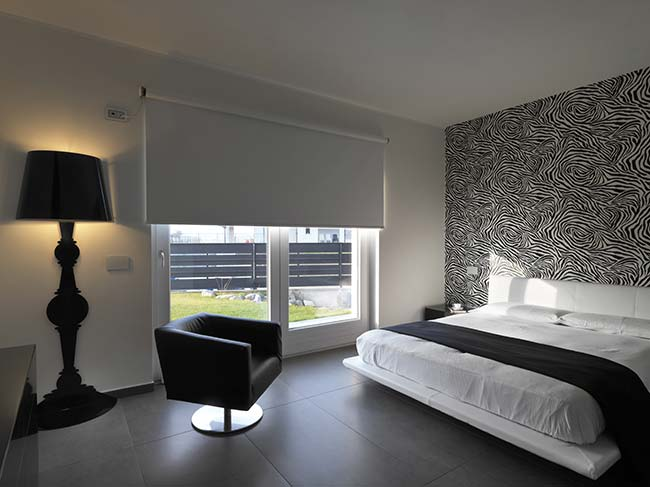### Budget

You live in a hypercompetitive world and therefore you will be bombarded with choices of tiles. Do your calculation and check how much budget you can allocate to tiles. Drill down the budget space-wise because each room/space will need different types of tiles. When you have a clear drilled-down budget, you will not drift off-course and spend impulsively.

### Area to be covered

There are 2 parts here

• Windows & Doors: If you plan to cover walls, you will leave out the space for the windows and doors. Calculate the number of tiles accordingly
• Plumbing and Wiring: If you conceal everything, you may have to break the tiles, each time there is a fault underneath. Breaking tiles periodically can damage the structure of the walls/floors besides costing you money.

## Benefits of Using Tiles

• Can be put up at different places in a house
• Easy to clean
• Simple and affordable maintenance
• Keeps the temperature under control
• Affordable
• Variety of designs available
• Long life

## Type of Tiles

### Ceramic tiles

Durable, affordable and recommended for areas with low to medium foot traffic like living and drawing room at home.   Ceramic tiles are available in a range of prints and sizes for you to fit in any interior theme.

### Porcelain tiles

• Denser and stronger as compared to ceramic
• scratch and stain resistant.

### Vitrified tiles

• strong
• durable
• stain and moisture resistant
• Double charged vitrified tiles available

## Measuring Floor and Wall Dimension

Measuring dimensions accurately is one of the key steps in calculating the number of tiles required. Some simple steps to measure floor dimension are listed below:

• To calculate how many tiles are needed for a floor, you must calculate the total area of your floor.
• To calculate the total number of tiles needed for a room, you must calculate the total area of the floor + walls (up to the height where you want the tiles to be installed)
• To find square footage for tiles, you must measure the area of a room, wall, or ceiling that you are covering with tiles.
• Walls are generally rectangular and have windows or doors on them that should be factored into your calculation.
• So, what we need to do is first calculate the area of the entire wall, then subtract the area of the window or door that’s also on the wall

### For example: Measuring Room Dimensions for Tiles

Length x Breadth x Height of a room= 20ft X 18ft X 10ft

Measuring the floor dimension:

Length x Breadth = 20ft x 18ft=360sq.ft

Floor Dimension = 360 sq. ft. x 1.1 = 396 sq. ft.

Measuring dimension of ONE wall:

Length x Height = 20 ft. x 10 ft. - 200 sq. ft.

• Length of window = 4 ft.
• Height of window = 3 ft.

dimension of window = 4 ft. x 3 ft. = 12 sq. ft.

• Actual wall area requiring tiling = A – B = 200 sq. ft. – 12 sq. ft. = 188 sq. ft. Add wastage of 10%

### Dimension of wall = 188 sq. ft. x 1.1 = 206.8 sq. ft.

If you are planning to tile other walls, you must calculate in the same manner and add the area of each wall to arrive at the total.

### For Non-Rectangular Floors

Although rooms in most houses are generally rectangular, if you have designed an offbeat room, you may try the following to calculate the floor area:

#### Circular room

school geometry. Use Pi.r2 to calculate the floor area. Pi = 3.14 and r = radius (half the diameter of the room)

#### Irregular shapes

Try and break down the space into multiple areas that make geometric sense. An L-shaped floor can be divided into two rectangles, each measuring 8ft x4ft. The area of one rectangle would be 32 sq. ft. and the total area of the floor would be 32 sq. ft. + 32 sq. ft. = 64 sq. ft.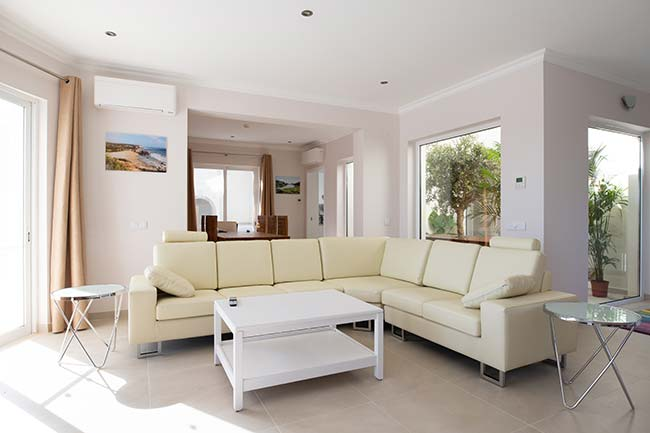## Selecting the Right Sized Tiles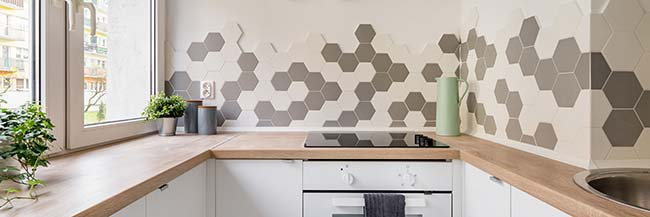Large tiles have a lesser number of joint lines and thus give an aura of spaciousness to the room. Select tiles for every space/location keeping this in mind:

• Optimise such that there is minimum wastage during installation.
• Pick bigger tiles for the living room, dining room and bedroom.
• Smaller tiles fit best in the kitchen and bathroom

### How Many Tiles Do You Need?

Once you have selected tiles for each of the rooms or spaces, how do you finally calculate the number of tiles required for each room?

• Keep the dimensions of each of the spaces ready
• Note the dimension of the tile for the respective space
• Divide the total area of the room/space by the total area of the tile that you have chosen for that room. For example, if the size of the tile is 1 sq. ft., you will need 120 tiles to cover a space of 120 sq. ft. If a box of tiles contains 10 tiles each, you will have to buy 12 such boxes.
• Keep a buffer. You should never buy the exact number of tiles basis your calculation. You must factor in losses during transportation and installation.

## Using a Tile Calculator

The calculator uses the same math described above. Divide the area of the room/floor/wall by the area of one tile. Just ensure you use round figures because you can’t buy tiles in fractions.

number of tiles = (area of space)/ (area of 1 tile)

The margin of error, i.e., damage during transportation and installation, is also factored in, in most of the quality tile calculators. Those extra buffers should be counted when calculating the number of boxes needed.

To keep the calculations simple, most calculators presume tiles are rectangular. If you are using other shapes, you must fill the gaps and that is where the buffer tiles would come in handy.

## Pro Tips

• Always keep extra tiles. Even if there is no damage at the time of installation, you may need them in the future. If you want to replace 1-2 tiles in future, you may not get the exact pattern, shape or colour.
• The calculations shown above in this article apply to any shape or pattern—straight, diagonal, diamond, etc. Keep an extra 15% in the total area to accommodate a fancy shape or an offbeat pattern.

## How Many Tiles are There in a Box?

Tiles are available in a variety of sizes. For floors, some popular one’s sizes include:

• 300 mm × 300 mm
• 600 mm × 600 mm
• 800 mm × 800 mm
• 1200 × 200 mm
• 1200 x 600 mm
• 1600x800
• 1800 x 1200 mm

Wall tiles are generally available in:

• 240 × 75 mm
• 300 × 450 mm
• 300×600 mm

## Cost of Tiles

Tile is an important constituent used in the construction of modern homes. The price of tiles varies considerably depending on your location, type (material), and quantity you buy. You can buy tiles starting from Rs 25 per sq. ft. and can go up to several hundred rupees.

### Typical Home Tiles

 Tile Type Rate per sq. ft. Ceramic tile Rs 30 to Rs 250 Porcelain tile Rs 25 to Rs 180 Vitrified tile Rs 60 to Rs 200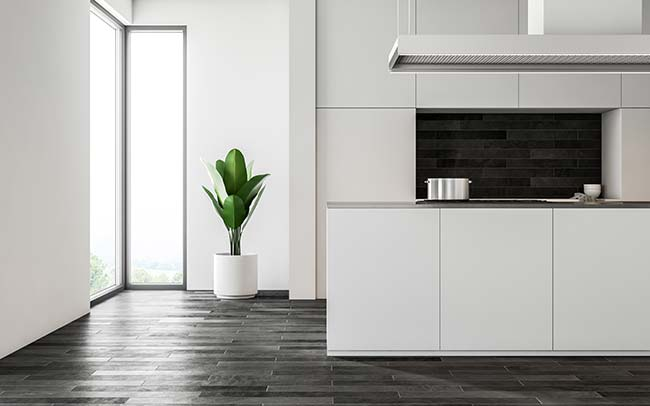## Typical Bathroom Tiles

 Tile Type Rate per sq. ft. Ceramic tile Rs 30 - Rs 65 Wooden tiles Rs 40 - Rs 125 Porcelain tiles Rs 65 - Rs 70 Vinyl tiles Rs 80 - Rs 160 Marble tiles Rs 90 - Rs 250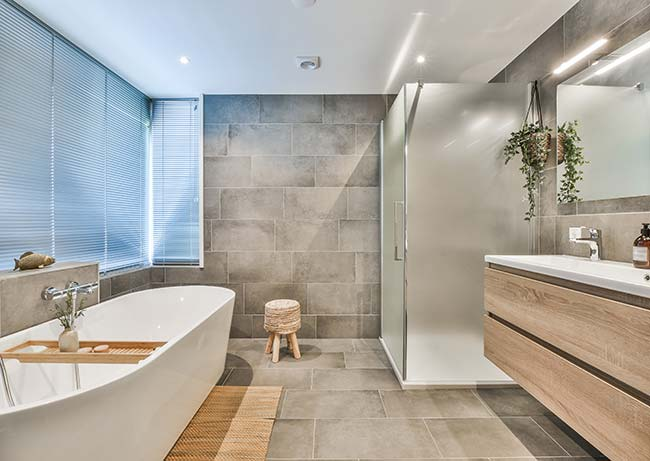Choosing the right number of tiles for your home will require some detailed study of the type, quality, design, size, pattern, and cost of tiles. Once you have these details and have made up your mind on the tile that you wish to install in each of the areas in your house, you can proceed to calculate the number of tiles needed.

Calculating the number of tiles can be done using a simple formula or by using the tile calculator. If you spend some time evaluating tiles and studying them more closely, you will be able to make an informed choice when purchasing tiles for your house. What’s more, you can even use tile-visualisation tools online to digitally view how your home would look with the new set of tiles.

Transform your home with new tiles. At the same time, order the right number of tiles so that you don’t run out of tiles when you need them.

### How do you calculate tile area?

You can calculate the area of the tile by using the tile calculator or the following formula.Divide the room/floor/wall area by the area of one tile. You must use round figures because fractional tiles are not available.

Area= Length x Width
For example: Area = l x w = 30 x 20 = 600 metre sq.

To keep the calculations simple, most calculators presume tiles are rectangular. If you are using other shapes, you must fill the gaps and where the buffer tiles would come in handy.

Most quality tile calculators account for the margin of error, which includes damage during transportation and installation. While calculating the number of boxes required, those extra buffers should also be considered.

For more information on tile area estimates kindly refer to the H&R Johnson Tile Guide

### How to calculate the tiles needed for a floor?

You can calculate how many tiles will be required if you are planning the installation. You need to follow some simple steps:

Step 1: For calculating how many tiles will be used on the floor, you must calculate the total area of your floor.

Step 2: The total area of the floor + walls must be calculated to determine the total number of tiles required for a room (up to the height where you want the tiles to be installed).

Step 3: The floor space of the project area is calculated by multiplying the length by the width.

Step 4: Tile is found commonly in boxes, and you must purchase the entire box. Calculate the total square footage of the room by dividing it by the total floor space of the tile in the box.
For example: If each tile is 1 square foot, you will need 120 tiles to conceal a 120 square foot area. You will need to purchase 12 boxes of tiles if each box contains 10 tiles.

Step 5: Keep the tiles buffer. You should never buy the exact number of tiles based on your calculation. You must factor in losses during transportation and installation.

For more information on tile quantity estimates kindly refer to the H&R Johnson Tile Guide

### How do you calculate tiles per square metre?

The first step is to measure your available space. You can do this yourself with a tape measure, or if you're unsure or have a more difficult area to tile, ask your tiler to do it for you. When we need a square metre measurement we are required to have that measure.

For example: A 60cm x 60cm tile fits 2.77 times into a square metre.
The most popular tile sizes are:

 Tile Sizes Number of Tiles per Square Metre 10cm x 20cm 50 Tiles 10cm x 30cm 33.33 Tiles 10cm x 60cm 16.67 Tiles 15cm x 15cm 44.44 Tiles 15cm x 30cm 22.22 Tiles

For more information on tile quantity estimates kindly refer to the H&R Johnson Tile Guide